• opencv对Mat进行排序

千次阅读 2018-11-13 11:02:16
由于sort函数和sortId函数是对一行或一列进行排序的，所以对整个需要reshape一下，还要指定是行还是列。 Mat c1 = (Mat_&lt;uchar&gt;(3, 3) &lt;&lt; 1, 5, 6, 2, 4, 3, 8, 9, 7); Mat c3 = c1...

由于sort函数和sortId函数是对一行或一列进行排序的，所以对整个需要reshape一下，还要指定是行还是列。

Mat c1 = (Mat_<uchar>(3, 3) << 1, 5, 6, 2, 4, 3, 8, 9, 7);
Mat c3 = c1.reshape(1, 9);
cv::sort(c3, c3, SORT_EVERY_COLUMN + CV_SORT_ASCENDING);

sortid返回是大小的索引

Mat c1 = (Mat_<uchar>(3, 3) << 1, 5, 6, 2, 4, 3, 8, 9, 7);
Mat c2(c1);
//sort(c2, c2, CV_SORT_EVERY_ROW + CV_SORT_ASCENDING);
sortIdx(c1, c2, SORT_EVERY_COLUMN + SORT_ASCENDING);

展开全文• OpenCV 之 矩阵排序(Mat)

千次阅读 2017-05-08 23:00:23
//mat_src：需要排序Mat类型的矩阵变量 mat_src.reshape(1,1).copyTo(mat_dst); cv::sort(mat_dst, mat_dst, CV_SORT_EVERY_ROW + CV_SORT_ASCENDING); cout << mat_dst ; 分析： reshape()函数 a. Mat cv::M

代码：

Mat mat_dst; //mat_src：需要排序的Mat类型的矩阵变量
mat_src.reshape(1,1).copyTo(mat_dst);
cv::sort(mat_dst, mat_dst, CV_SORT_EVERY_ROW + CV_SORT_ASCENDING);
cout << mat_dst << endl;

分析：

1. reshape()函数
a. Mat cv::Mat::reshape( int cn, int rows = 0 )
OpenCV官方讲解得非常清楚：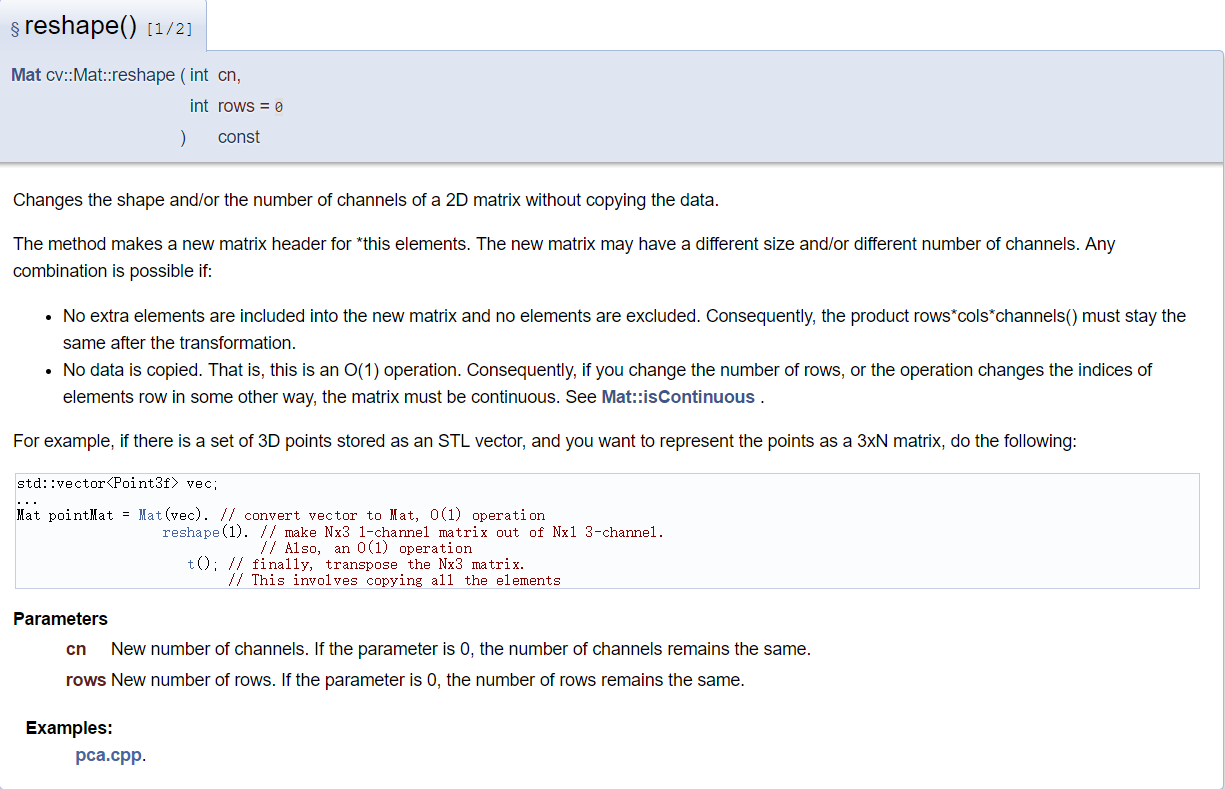b. Mat cv::Mat::reshape( int cn, int newndims, const int * newsz )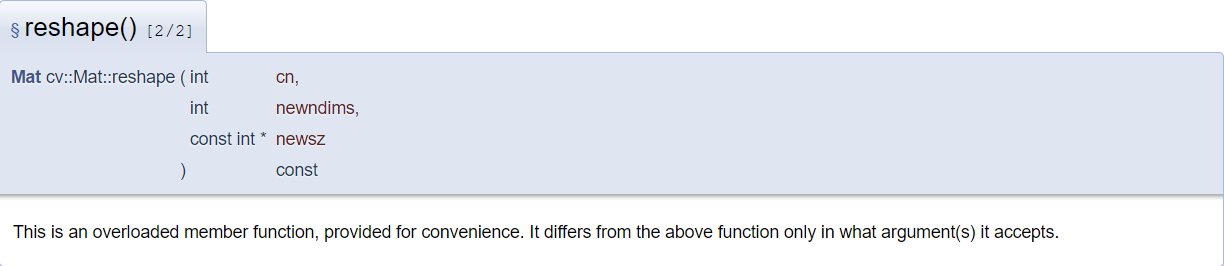c. Examples
可参考：http://docs.opencv.org/3.2.0/d2/dc0/pca_8cpp-example.html#a17

2. sort()函数
OpenCV官方介绍：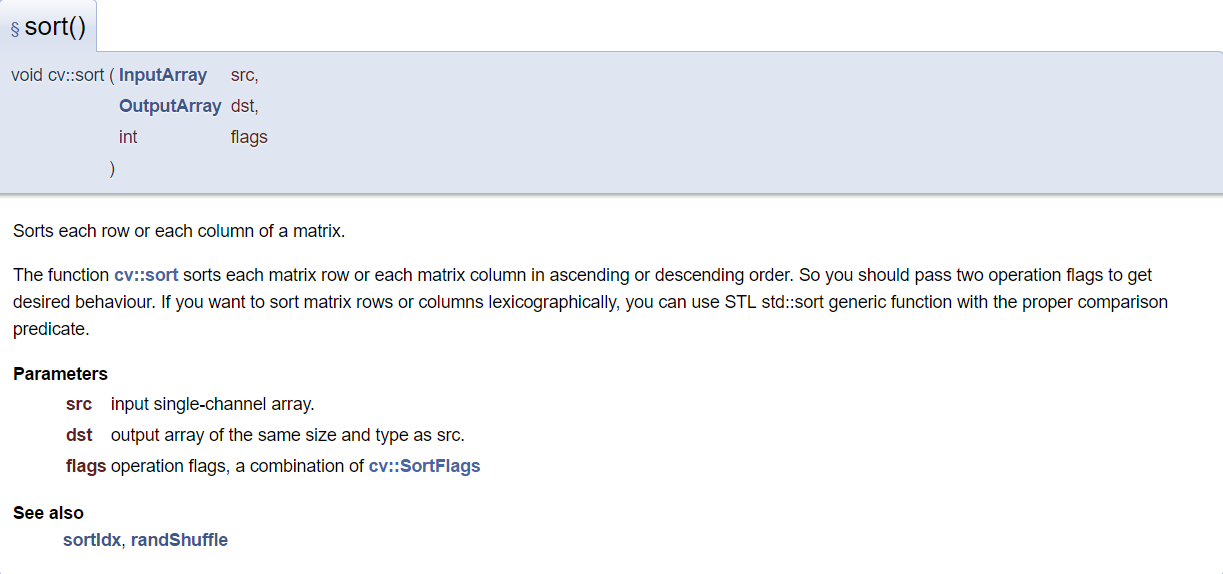相关函数：
void cv::sortIdx( InputArray src, OutputArray dst, int flags )；

展开全文opencv Mat 排序
• //根据指定列以行为单位排序 Mat sorted_index; cv::sortIdx(stats, sorted_index, cv::SORT_EVERY_COLUMN + cv::SORT_DESCENDING); // 注意此处是DESCENDING 如果要升序需要改成ASCENDING sorted_index = ...
void sortMat(Mat &stats, int colId){
//根据指定列以行为单位排序

Mat sorted_index;
cv::sortIdx(stats, sorted_index, cv::SORT_EVERY_COLUMN + cv::SORT_DESCENDING);
// 注意此处是DESCENDING 如果要升序需要改成ASCENDING

sorted_index = sorted_index.col(colId);
Mat sorted_stats = stats.clone();
int row_num = sorted_index.rows;
for(int i = 0; i < row_num; i++){
int _idx = sorted_index.at<int>(i, 0);
sorted_stats.row(i) = stats.row(_idx) + 0;//必须加0否则会出很难debug的错误
}
stats = sorted_stats;
return;
}

展开全文• 原文：http://blog.csdn.net/qing101hua/article/details/52817373 sortIdx 函数 对元素进行排序， 返回对应的排序索引 ...Mat c1 = (Mat_double>(3,3) Mat c2(c1); sortIdx(c1, c2, SO

原文：http://blog.csdn.net/qing101hua/article/details/52817373

sortIdx 函数 对元素进行排序， 返回对应的排序索引

1. Mat c1 = (Mat_<double>(3,3) << 1, 5 , 6 , 2 , 4, 2, 5, 9, 4);
2. Mat c2(c1);
3. sortIdx(c1, c2, SORT_EVERY_COLUMN + SORT_ASCENDING);
4. cout << "c1: \n" << c1 << endl;
5. cout << "c2: \n" << c2 << endl;
Mat c1 = (Mat_<double>(3,3) << 1, 5 , 6 , 2 , 4, 2, 5, 9, 4);
Mat c2(c1);
sortIdx(c1, c2, SORT_EVERY_COLUMN + SORT_ASCENDING);
cout << "c1: \n" << c1 << endl;
cout << "c2: \n" << c2 << endl;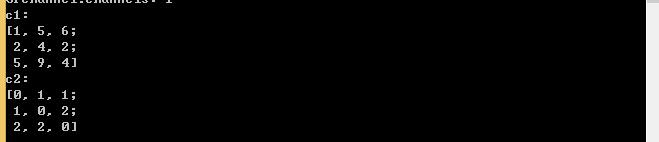sort 排序函数， C++中sort函数 详细介绍参见 ：  http://blog.csdn.net/qing101hua/article/details/52822060

1. // sort  Mat(3,3)
2. int* c1begin = c1.ptr<int>(0);
3. int* c1end = c1.ptr<int>(2);
4. sort(c1begin, c1end); // 该行代码 编译报错
5. cout << "c1: \n " << c1 << endl;
// sort  Mat(3,3)
int* c1begin = c1.ptr<int>(0);
int* c1end = c1.ptr<int>(2);
sort(c1begin, c1end); // 该行代码 编译报错
cout << "c1: \n " << c1 << endl;

1>C:\Program Files (x86)\Microsoft Visual Studio 12.0\VC\include\algorithm(3093): error : operand of "*" must be a pointer
1>C:\Program Files (x86)\Microsoft Visual Studio 12.0\VC\include\algorithm(3093): error : operand of "*" must be a pointer
1>C:\Program Files (x86)\Microsoft Visual Studio 12.0\VC\include\algorithm(3093): error : no instance of function template "std::less<void>::operator()" matches the argument list
1>C:\Program Files (x86)\Microsoft Visual Studio 12.0\VC\include\algorithm(2288): error : operand of "*" must be a pointer
1>C:\Program Files (x86)\Microsoft Visual Studio 12.0\VC\include\algorithm(2288): error : operand of "*" must be a pointer
1>C:\Program Files (x86)\Microsoft Visual Studio 12.0\VC\include\algorithm(2292): error : operand of "*" must be a pointer
1>C:\Program Files (x86)\Microsoft Visual Studio 12.0\VC\include\algorithm(2292): error : operand of "*" must be a pointer
1>  main.cu
1>C:\Program Files (x86)\MSBuild\Microsoft.Cpp\v4.0\V120\BuildCustomizations\CUDA 7.5.targets(604,9): error MSB3721: 命令“"C:\Program Files\NVIDIA GPU Computing Toolkit\CUDA\v7.5\bin\nvcc.exe" -gencode=arch=compute_20,code=\"sm_20,compute_20\" --use-local-env --cl-version 2013 -ccbin "C:\Program Files (x86)\Microsoft Visual Studio 12.0\VC\bin\x86_amd64"  -I"C:\Program Files\NVIDIA GPU Computing Toolkit\CUDA\v7.5\include" -I"C:\Program Files\NVIDIA GPU Computing Toolkit\CUDA\v7.5\include"  -G   --keep-dir x64\Debug -maxrregcount=0  --machine 64 --compile -cudart static  -g   -DWIN32 -DWIN64 -D_DEBUG -D_CONSOLE -D_MBCS -Xcompiler "/EHsc /W3 /nologo /Od /Zi /RTC1 /MDd " -o x64\Debug\main.cu.obj "D:\work\test\wb\wb\main.cu"”已退出，返回代码为 2。
========== 全部重新生成:  成功 0 个，失败 1 个，跳过 0 个 ==========

1. //sort int;
2. int d1[] = { 2, 4, 5, 2, 1, 8, 6, 7, 9 };
3. sort(d1, d1);   // 报错 ，编译不过去
4. //cout << "d1: \n" << d1 << endl;
//sort int;
int d1[] = { 2, 4, 5, 2, 1, 8, 6, 7, 9 };
sort(d1, d1);   // 报错 ，编译不过去
//cout << "d1: \n" << d1 << endl;

报错：

1>C:\Program Files (x86)\Microsoft Visual Studio 12.0\VC\include\algorithm(3093): error : no instance of function template "std::less<void>::operator()" matches the argument list
1>C:\Program Files (x86)\Microsoft Visual Studio 12.0\VC\include\algorithm(2288): error : operand of "*" must be a pointer
1>C:\Program Files (x86)\Microsoft Visual Studio 12.0\VC\include\algorithm(2288): error : operand of "*" must be a pointer
1>C:\Program Files (x86)\Microsoft Visual Studio 12.0\VC\include\algorithm(2292): error : operand of "*" must be a pointer
1>C:\Program Files (x86)\Microsoft Visual Studio 12.0\VC\include\algorithm(2292): error : operand of "*" must be a pointer
1>  main.cu
1>C:\Program Files (x86)\MSBuild\Microsoft.Cpp\v4.0\V120\BuildCustomizations\CUDA 7.5.targets(604,9): error MSB3721: 命令“"C:\Program Files\NVIDIA GPU Computing Toolkit\CUDA\v7.5\bin\nvcc.exe" -gencode=arch=compute_20,code=\"sm_20,compute_20\" --use-local-env --cl-version 2013 -ccbin "C:\Program Files (x86)\Microsoft Visual Studio 12.0\VC\bin\x86_amd64"  -I"C:\Program Files\NVIDIA GPU Computing Toolkit\CUDA\v7.5\include" -I"C:\Program Files\NVIDIA GPU Computing Toolkit\CUDA\v7.5\include"  -G   --keep-dir x64\Debug -maxrregcount=0  --machine 64 --compile -cudart static  -g   -DWIN32 -DWIN64 -D_DEBUG -D_CONSOLE -D_MBCS -Xcompiler "/EHsc /W3 /nologo /Od /Zi /RTC1 /MDd " -o x64\Debug\main.cu.obj "D:\work\test\wb\wb\main.cu"”已退出，返回代码为 2。
========== 生成:  成功 0 个，失败 1 个，最新 0 个，跳过 0 个 ==========

解决：

将sort输入参数 改成数据索引的指针

1、对int 进行测试， 对int的前6个元素进行排序

1. //sort int;
2. int d1 = { 2, 4, 5, 2, 1, 8, 6, 7, 9 };
3. cout << "d1[]: " << endl;
4. for (int i = 0; i < 9; i++)
5.  cout << d1[i] << endl;
6.
7. sort(d1, d1+5);   // 注意： 参数如果不是指针变量的话 会报错 ，编译不过去
8. cout << "d1[]: " << endl;
9. for (int i = 0; i < 6; i++)
10.  cout << d1[i] << endl;
//sort int;
int d1 = { 2, 4, 5, 2, 1, 8, 6, 7, 9 };
cout << "d1[]: " << endl;
for (int i = 0; i < 9; i++)
cout << d1[i] << endl;

sort(d1, d1+5);   // 注意： 参数如果不是指针变量的话 会报错 ，编译不过去
cout << "d1[]: " << endl;
for (int i = 0; i < 6; i++)
cout << d1[i] << endl;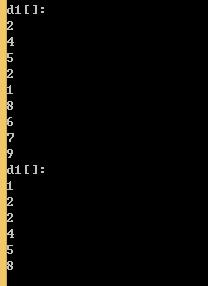2、对Mat（3,3）的元素进行排序：

1. int* c1begin = c1.ptr<int>(0);
2. int* c1end = c1.ptr<int>(2);
3. sort(c1begin, c1end+2);
4. cout << endl<<"sort(c1begin, c1end+2)" << endl;
5. cout << "c1: \n " << c1 << endl;
6.
7. sort(c1begin, c1end + 3);
8. cout <<endl << "sort(c1begin, c1end+3)" << endl;
9. cout << "c1: \n " << c1 << endl;
int* c1begin = c1.ptr<int>(0);
int* c1end = c1.ptr<int>(2);
sort(c1begin, c1end+2);
cout << endl<<"sort(c1begin, c1end+2)" << endl;
cout << "c1: \n " << c1 << endl;

sort(c1begin, c1end + 3);
cout <<endl << "sort(c1begin, c1end+3)" << endl;
cout << "c1: \n " << c1 << endl;
1. sort(c1begin, c1end);
2. cout << endl << "sort(c1begin, c1end)" << endl;
3. cout << "c1: \n " << c1 << endl;
sort(c1begin, c1end);
cout << endl << "sort(c1begin, c1end)" << endl;
cout << "c1: \n " << c1 << endl;

展开全文矩阵 函数 opencv
• 2、对Mat（3,3）的元素进行排序： int* c1begin = c1.ptr(0); int* c1end = c1.ptr(2); sort(c1begin, c1end+2); cout (c1begin, c1end+2)" ; cout ; sort(c1begin, c1end + 3); cout (c1begin, c1...
• 角材料垫表 该项目是使用版本9.1.10生成的。 开发服务器 为开发服务器运行ng serve 。... 如果您更改任何源文件，该应用程序将自动重新加载。 代码脚手架 运行ng generate component component-name生成一个新的组件。...TypeScript
• mat4py-以Matlab（MATMAT... 数字和单元格数组将转换为按行排序的嵌套列表。 压缩数组以消除仅包含一个元素的数组。 结果数据结构由与JSON格式兼容的简单类型组成。 示例：将MAT文件加载到Python数据结构中： daPython
• Is there an equivalent function in OpenCV similar to [srtd,srtdinds] = sort(dst,'ascend'); in Matlab? I have tried cv::sortIdx(source, dst, cv::SORT_ASCENDING);... My source Mat cont...cv sort函数
• MAT使用

2021-08-09 00:06:29
MAT使用 Eclipse Memory Analyzer 使用 设置 1.运行时堆内存配置 /Applications/mat.app/Contents/Eclipse/MemoryAnalyzer.ini -Xmx1024m 2.选项配置 ![[Pasted image 20210804140711.png]] Keep unreachable ...
• MAT简介MAT是一款非常强大的内存分析工具，在Eclipse中有相应的插件，同时也有单独的安装包。在进行内存分析时，只要获得了反映当前设备内存映像的hprof文件，通过MAT打开就可以直观地看到当前的内存信息。一般说来...java mat类型
• 分析类加载器 四、MAT使用介绍 4.1 Histogram直方图 Histogram是使用最多的一个，可以列出内存中的对象，对象的个数及其大小 4.1.1 具体信息 具体信息 Class Name ： 类名称，java类名 Objects ： 类的对象的数量，...java JVM
• 一定要注意mat版本，用MemoryAnalyzer-1.4.0.20140604-win32.win32.x86版本才可以。其他版本估计要依赖于高版本的jdkEclipse Memory Analyzer Tool(MAT)是一个强大的基于Eclipse的内存分析工具，可以帮助我们找到...
• 一、冒泡排序原理解析：(以从小到大排序为例)在一排数字中，将第一个与第二个比较大小，如果后面的数比前面的小，则交换他们的位置。然后比较第二、第三个……直到比较第n-1个和第n个，此时，每一次比较都将较大的一...
• MAT使用说明

千次阅读 2018-12-26 17:06:32
第一次打开文件MAT进行分析处理会比较慢。 打开以后会展示如下效果 上图中的几个主要信息： Details: 堆内存大小(Size)、类的数量(Classes)、对象的数量(Objects)、类加载器的数量(Class Loader)。 Biggest ...MAT使用
• 1内存泄漏的排查方法Dalvik Debug Monitor Server (DDMS)是ADT插件的一部分，其中有两项功能可用于内存检查:·heap查看堆的分配情况·allocation ...Eclipse Memory Analysis Tools (MAT)是一个分析Java堆数据的专业...mat 释放
• MAT 使用教程

千次阅读 2018-07-02 10:01:55
MAT 使用教程 Memory Analysis Tools (MAT) 是一个分析 Java 堆数据的专业工具，用它可以定位内存泄漏的原因。 观察 Heap 运行程序，然后进入 DDMS管理界面，如下： 点击工具栏上的 来更新统计信息 点击...mat 内存泄露
• 前面介绍过用dnarray来模拟，但mat更符合矩阵，这里的mat与matlab中的很相似。(mat与matrix等同)基本操作>>> m= np.mat([1,2,3]) #创建矩阵>>> mmatrix([[1, 2, 3]])>>> m #取一行...
• 1.MAT简介：jvm内存溢出/内存泄漏问题分析定位神器MAT 全称Eclipse Memory Analysis Tools 是一个分析 Java堆数据的专业工具，可以计算出内存中对象的实例数量、占用空间大小、引用关系等，看看是谁阻止了垃圾收集器...mat 释放
• 又不允许随便破坏环境，更通常的做法是，导出日志，利用第三方工具进行排查 本篇将针对这一点，简单介绍下一款强大的dump日志分析工具，Eclipse Memory Analyzer，也称作MAT MAT是什么 MAT工具是一款强大的Java堆...mat使用
• Android应用开发过程中，...而MAT就是一款非常好用的分析应用内存使用情况的工具，使用起来也非常简单，那么本文就详细介绍android内存分析工具Mat。1 内存泄漏的排查方法Dalvik debug Monitor Server (DDMS) 是 ...
• MAT使用教程

万次阅读 2015-09-27 00:36:16
MAT 全称 Eclipse Memory Analysis Tools 是一个分析 Java堆数据的专业工具，可以计算出内存中对象的实例数量、占用空间大小、引用关系等，看看是谁阻止了垃圾收集器的回收工作，从而定位内存泄漏的原因。...
• 第一篇文章《Android內存優化之一：MAT使用入門》介紹了MAT的基本使用方法，包括下載、安裝、打開HPROF文件，和一些基本的視圖。這篇文章介紹一下一些最新的工具使用方法，和第一篇中沒有提到的一些其他的用法。Java...
• 1内存泄漏的排查方法Dalvik Debug Monitor Server (DDMS)是ADT插件的一部分，其中有两项功能可用于内存检查:·...Eclipse Memory Analysis Tools (MAT)是一个分析Java堆数据的专业工具，用它可以定位内存泄漏的原...
• clc 清除指令窗 clear 清除内存变量和函数 clf 清除图对象 clock 时钟 colorcube 三浓淡多彩交叉色图矩阵 colordef 设置色彩缺省值 colormap 色图 colspace 列空间的基 close 关闭指定窗口 colperm 列排序置换向量 ...
• 根据hash_map的val值排序(求众数) Step1:将数据传入mp中，并进行计数； Step2:定义一个vector<pair<int, int>>temp，并把mp中的数据拷贝到temp中； Step3:根据pair的second进行排序，即对每个数出现...哈希 排序
• np.mat()函数与np.array()函数的辨析

千次阅读 多人点赞 2020-02-07 16:22:27
写在前面 今天，在学习numpy的时候，偶然看到np.mat()函数，查了一下，也是生成矩阵，这里的mat与MATLAB中的很相似，所以在这里简单的记录一下np.mat...排序等 >>> m= np.mat([1,2,3]) #创建矩阵 >>...numpy python mat函数 array函数
• Android Studio和MAT结合使用来分析内存问题

万次阅读 多人点赞 2016-08-09 22:39:44
Android开发中时常会遇到内存泄漏的问题，而Android系统对单个App又有一定的内存限制，此值可以通过一下方式...Android studio自带的内存分析工具直观方便，但其功能却不如MAT强大，特别是没有有效的搜索、排序等功能。...内存泄漏 内存优化
• JVM系列文章目录 初识JVM 深入理解JVM内存区域 玩转JVM对象和引用 JVM分代回收机制和垃圾回收算法 细谈JVM垃圾回收与部分底层实现 ...玩转MAT分析内存泄漏JVM系列文章目录前言MAT概要柱状视图MAT内存泄漏分析java jvm
• 答案 2 :(得分：0) 我根据唯一标识符将bigmat拆分为块，然后重新排序块，如下所示： %# this assumes bigmat is sorted, btw %# i.e. that the different ids are grouped together %# find unique ids dd = [true;...
• MAT助手是一款专门为mat用户打造的手机软件，用户可以使用这款软件对应用包的文件名进行修改，对于mat用户来说绝对是一个小巧而实用的神器，操作也特别简单，小白用户勿入，需要的可以来西西下载MAT助手进行体验MAT......